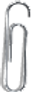## Essestial Questions

1.  What are the components of a full description of an object’s motion?

2.  How are position, velocity, and acceleration related?

## Essential Standard

PSc.1.1 Understand motion in terms of speed, velocity, acceleration, and momentum.

## Clarifying Objective

PSc.1.1.1 Explain motion in terms of frame of reference, distance, and displacement.

PSc.1.1.2 Compare speed, velocity, acceleration, and momentum using investigations, graphing, scalar quantities and vector quantities.

Position

Displacement

Distance

Velocity

Speed

Acceleration

Momentum

## Interactive Notes